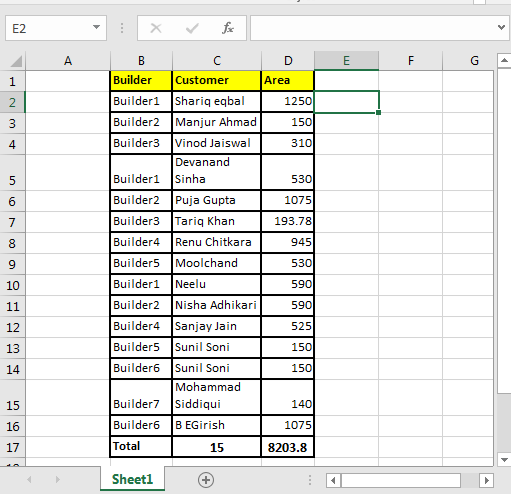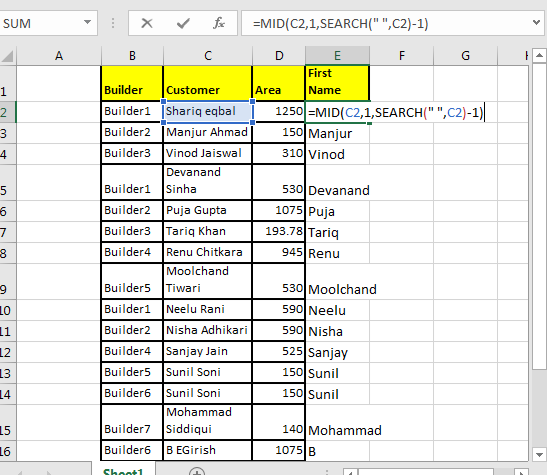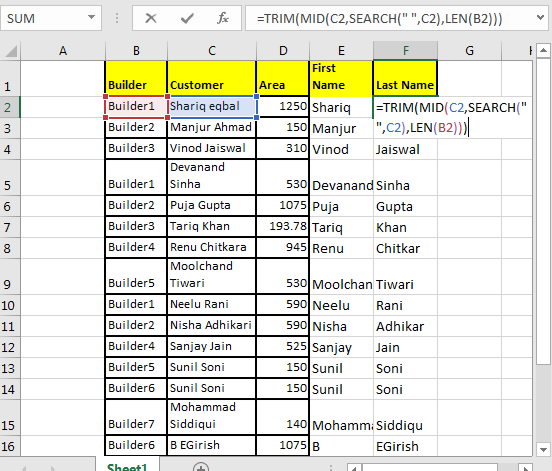# The MID Function in Excel

The Excel MID function is used to extract text from the middle of the string. Basically n number of characters from a given index in the string.Syntax

=MID(text, start_num, num_of_chars)

MID Function Example
If you know from which position how many characters are needed to extract simply use the MID function.

The below formula will extract city code 110094.

=MID(“AVD110094Delhi”,4,6)

Here text is “AVD110094Delhi”.
Start_num is 4 since city code starts from 4th position.
Num_of_chars is 6 because the city code is 6 characters long.
Here, we knew how many characters are there and from which position they start. What if we don’t know this? Next example illustrates another amazing use of MID function.
Extract First Name Using Excel MID Function
Generic Formula to Extract First Name in Excel 2016

=MID(text,1,SEARCH(“ “,text)-1)

I have this table. Now, I want to extract first name from column C.To extract first name write this MID formula and drag it down:

=MID(C2,1,SEARCH(" ",C2)-1)Here, we knew the starting point (start_num) for first name but didn’t knew the number of characters in first name.
So we took SEARCH function’s help to find the “space” (“ ”) in text.
Then we subtracted 1 to exclude that space from extracted text. Simple.
Extract Last Name Using Excel MID Function
Generic Formula to Extract Last Name in Excel 2016

=TRIM(MID(C2,SEARCH(" ",C2),LEN(B2)))
=TRIM(MID(C2,SEARCH(" ",C2),LEN(B2)))Here we don’t know the start_num nor the num_chars.

To find start_num we took help of the SEARCH function.
We don’t know the number of characters to extract. And we don’t need to. We will just use the LEN function. It will give the length of the string. Hence it will return all the characters remaining after start_num.

TRIM is used to delete the space before the text.

So, yeah! The MID function is simply a text extraction function. But as they say, a sword is as powerful as a knight.

Related Articles:

How to use RIGHT function in Excel

Left Function in Microsoft Excel

Split Numbers and Text from String in Excel

Extract Text From A String In Excel Using Excel’s LEFT And RIGHT Function

Popular Articles:

50 Excel Shortcuts to Increase Your Productivity

How to use the VLOOKUP Function in Excel

How to use the COUNTIF function in Excel 2016

How to Use SUMIF Function in Excel

Terms and Conditions of use

The applications/code on this site are distributed as is and without warranties or liability. In no event shall the owner of the copyrights, or the authors of the applications/code be liable for any loss of profit, any problems or any damage resulting from the use or evaluation of the applications/code.